# Magic Letters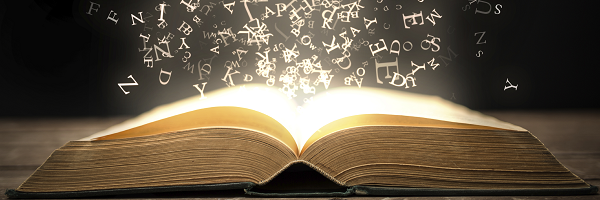Charlie has made a Magic V with five consecutive numbers: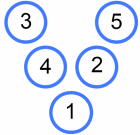It is a Magic V because each 'arm' has the same total.

Alison drew this magic V: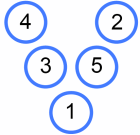Charlie said "That's really just the same Magic V as mine!"

What do you think Charlie meant?
There are six more Magic Vs that are the same as Charlie's - can you find them all?

Click below to check you have.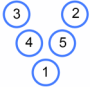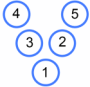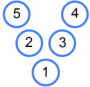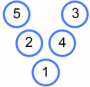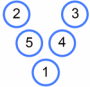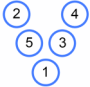Can you find all the possible Magic Vs that use the numbers $1$ to $5$ and are different from Charlie's?

How can you be sure you have found them all?

What happens if you use the numbers from $2$ to $6$? Or from $3$ to $7$? Or $4$ to $8\dots$?

Can you describe an efficient strategy to find all Magic Vs for any given set of numbers?

Can you use your strategy to find all the possible Magic Vs that use the numbers $987, 988, 989, 990, 991$?

Can you find a Magic V where each arm has a total of 60? Or 1000? Or...?
Can you find more than one?
Can you find a Magic V for any arm total?

Charlie and Alison drew some more letters, to see if they could make them magic - that is, using consecutive numbers to make equal arm totals.

 Magic L? Magic N? Magic W?Investigate some of these Magic Letters in the same way that you explored Magic Vs.
What general conclusions can you reach?

You can use this spreadsheet to explore.

### Magic Letters

Alison's magic V was the same as Charlie's, because Alison had just swapped the 5 and the 2, and the 4 and the 3, around so it looked different, but really was exactly the same. The 'arms' still added up to 8.
It was as if you copied someone but just did 4+3+1 and 2+5+1 instead of 3+4+1 and 5+2+1. Exactly the same.

### Pattern and structures

For question 1 there are no other Magic V's containing the numbers 1 to 5 that are different from Charlie's, because you can only mix the top 4 number across and down 4 times, so if you do 4 x 2 sides it equals 8 and the eight Magic V's have already been drawn.

### Patterns and structures

But could you find a different Magic V containing the numbers 1 to 5 which didn't have a 1 at the bottom?

### The strategy

You do the number of odd numbers times 8 if there are more odds than evens and you do the number of evens times 8 if there are more evens than odd, the bottom of the v has to be either odd or even so that the 2 arms of the v contain an odd and an even number and it balances out. If there are 3 odds, 2 evens, bottom of the v is odd so it goes odd even - odd - odd even and the arms can mix around 8 times, it's the other way round for more evens.

### Magic v

You put the lowest number in the box at the bottom then use the highest and second lowest in one side and use the rest of the numbers on the other side

### Strategy for Making a Magic V

You can easily make a Magic V from 5 consecutive numbers because you just do the following:
Use the 1st, 3rd or 5th number as the bottom point.
For the 4 numbers left over, pair the biggest one with the smallest and pair the two in between.
These pairs would make arms of the same value.
For example:
5 consecutive numbers: 7, 8, 9, 10, 11.
For the sake of argument - the third number (9) is used as the bottom point.
Biggest number (11) paired with smallest number (7). Arm = 11 + 7 = 18.
Two numbers in between (10 & 8) paired. Arm = 10 + 8 = 18.

### New solution

I found 6 magic vs. 3 of which aren't on your answers. It was easy after I got the hang of it :)

Starting at the end of an arm and listing the numbers in order as I go down and up the other arm:

4, 3, 2, 5, 1
4, 3, 2, 1, 5
3, 4, 2, 5, 1

4, 3, 1, 2, 5
3, 4, 1, 5, 2
2, 5, 1, 3, 4

### New solution

Hi Joe,
I'm afraid your first three are not Magic Vs. The arms with 2, 3 and 4 add to 1 more than the arms with 2, 1 and 5.

The last three are all "the same" - they all have an arm with 1, 2 and 5 and another arm with 1, 3 and 4.

Can you find some different Magic Vs?

### 3 "Unique" Magic Vs

Say we have five consecutive numbers, a, a + 1, a + 2, a + 3, a + 4.
Now, we have five cases, where each of the five numbers is at the root of the Magic V.

The first case: a is the root of the V. Moving down one arm and up the other arm, we have the following unique Magic V (along with three identical Magic Vs): a + 1, a + 4, a, a + 2, a + 3. These four are the only solutions for the first case.

The second case: a + 1 is the root of the V. Unfortunately, there are no solutions for this case, because out of the remaining numbers, three are even and one is odd, or three are odd and one is even, and thus one of the arms will sum up to be an odd number and the other will sum up to be an even number.

The third case: a + 2 is the root of the V. Moving down one arm and up the other arm, we have the following unique Magic V (along with three identical Magic Vs): a, a + 4, a + 2, a + 1, a + 3. These four are the only solutions for the third case.

The fourth case: a + 3 is the root of the V. Unfortunately, there are no solutions for this case for the same reason as the second case.

The fifth case: a + 4 is the root of the V. Moving down one arm and up the other arm, we have the following unique Magic V (along with three identical Magic Vs): a + 1, a + 2, a + 4, a, a + 3. These four are the only solutions for the fifth case.

In total, there are twelve different possible Magic Vs for any five consecutive numbers, three of which are unique Magic Vs according to Charlie's bizarre redefinition of "same".### Hi Jan - sorry you think

Hi Jan - sorry you think Charlie's comment that the two Vs were the same was bizarre! Younger children may not have explored the idea of uniqueness and what it means for two solutions to be distinct, so it's just intended to be a way of starting up that conversation.

### A Magic V for any sum.

Nick Taylor, Brock Dyer, Saeed Nin, Matt Dominico, Zach Kline, Brian Ngov, Shane Vandegrift

Given any whole number $X$ greater than $6$, the following sequence will generate the Magic V that has the legs sum to $X$. The lowest Magic V leg sum created from five consecutive positive integers is $7$ where the digits would be $0$, $1$, $2$, $3$, and $4$. Any lower sum would require negative integers.

If $X$ is a multiple of $3$, then:
The vertex of the Magic V is $\frac{X}{3}$, called $V$.
The set of consecutive integers to generate the Magic V are $V - 2$, $V-1$, $V$, $V+1$, $V + 2$
Leg A is $V+1$ & $V-1$ $\qquad\qquad$ Leg B is $V+2$ & $V-2$

If $X$ is a non-multiple of $3$ and EVEN, then:
The median of the set of consecutive integers for the Magic V is found: $M = \frac{X+1}{3}$
The set of consecutive integers to generate the Magic V are $M - 2$, $M-1$, $M$, $M+1$, $M + 2$
The vertex is $M - 2$
Leg A is $M - 1$ & $M + 2$ $\qquad\qquad$ Leg B is $M$ & $M+1$

If $X$ is a non-multiple of $3$ and ODD, then:
The median of the set of consecutive integers for the Magic V is found: $M = \frac{X-1}{3}$
The set of consecutive integers to generate the Magic V are $M - 2$, $M-1$, $M$, $M+1$, $M + 2$
The vertex is $M + 2$
Leg A is $M + 1$ & $M - 2$ $\qquad\qquad$ Leg B is $M$ & $M-1$

Each Magic V has 8 rotations possible.

### A Magic V for any sum.

That's some excellent work, well done! Could you give some examples sums for the arms summing to 60 or 1000?

Could you use your ideas to develop ways to generate other magic letters, such as L's, N's or W's?